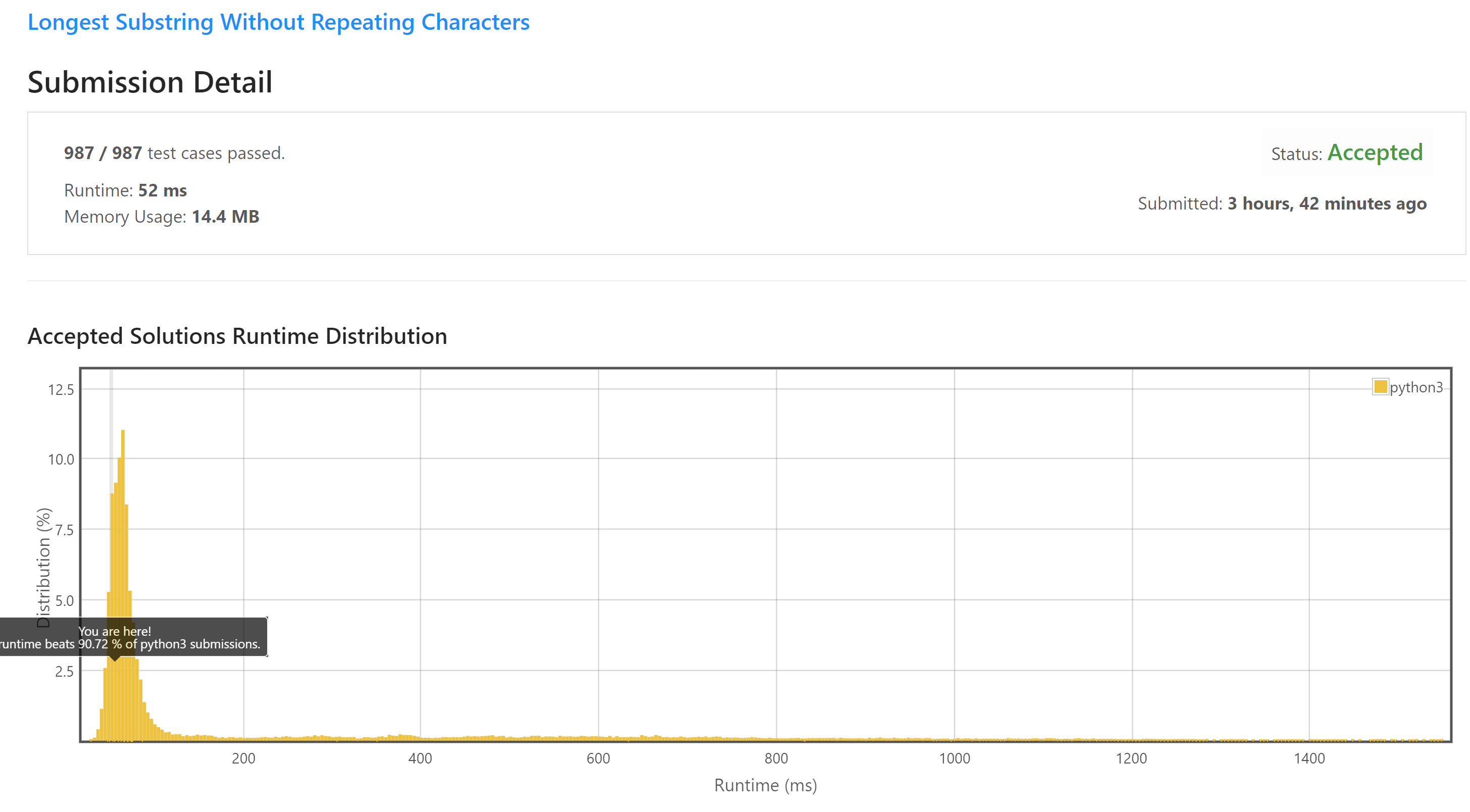I have been doing some interviews for job positions like data scientist, machine learning engineer, and software developer during the past months. To prepare for the coding part of these interviews and brush up on my algorithmic thinking and programming skills, I decided to do some ad-hoc practicing. There are lots of websites with coding challenges of varying difficulty. Some examples include Leetcode, HackerRank, Topcoder, and others. Although I kind of dislike the contrived nature of these quizzes, I joined Leetcode nonetheless. Anyway, I picked a problem under the “medium” difficulty category that I’ll blog about today. The problem is about finding the longest substring with non-repeating characters in a string.

### Problem formulation

Given a string s, find the length of the longest substring without repeating characters.

Example 1: Input: s = “abcabcbb” Output: 3 Explanation: The answer is “abc”, with the length of 3.

Example 2: Input: s = “bbbbb” Output: 1 Explanation: The answer is “b”, with the length of 1.

Example 3: Input: s = “pwwkew” Output: 3 Explanation: The answer is “wke”, with the length of 3. Notice that the answer must be a substring, “pwke” is a subsequence and not a substring.

Example 4: Input: s = “” Output: 0

Constraints: $$0 \le \text{s.length} \le 5 \times 10^4$$ s consists of English letters, digits, symbols and spaces.

### Solutions

We import some libraries that we will need later on.

For starters, we will write a function that generates random strings consisting of lowercase letters, digits, and whitespace characters of varying lengths. We will use it to see how our different solutions scale with increasing input size. When coding such problems, it’s essential to have abundant examples that cover all edge cases. By the way, I’ve found it easier to write and run my code in a Jupyter Notebook inside Visual Studio Code and then paste it to Leetcode.

### The horrible solution

My first attempt resulted in the following readable yet absolutely horrible, complexity-wise, solution. The rep() function is good, actually, and we will be using it in the other solutions as well. It uses a dictionary to track whether the next character has already been seen inside a substring. It has the advantage that it iterates only once the substring, so it’s $$\mathcal{O}(N)$$ time complexity. Had we used a nested loop to search for repeating characters, that would lead us to $$\mathcal{O}(N^2)$$ complexity from the get-go!

So, the following algorithm starts with the entire string and checks whether it has any repeating characters. If it doesn’t, then this is the longest substring of length N! Return its length, and we are done. If it has repeating characters, though, we slice it into two N-1 substrings. If the repeating characters are located in only one out of the two, we know that the other is the longest substring with a length N-1. Return it immediately, and we are done. Last, if there are repeating characters in both the substrings of length N-1, we need to dig deeper, and therefore we return the maximum length of the substring contained in these two substrings.

So, why does this algorithm perform so poorly? As I understand, there are two reasons: 1. Recursion is expensive because each time we call the helper() function, a new stack frame needs to be allocated, and 2. When we are calling max(helper(a, n-1), helper(b, n-1)), we don’t really divide the input, let alone conquer it! We merely go from N to N-1. It’s not as if we reduced the search space from N to N/2 or something. So, remember: if you recurse, you better be dividing the search space at each step, otherwise don’t recurse!

### A decent solution of $$\mathcal{O}(N^2)$$ complexity

The next two solutions use sliding windows, either forward or backward, to find all possible substrings in a string. The forward method keeps track of the length that is maximum at the current point in time. We need to do this because we must look up to the original string of length N.

On the other hand, if we are moving backward, i.e., if we are examining substrings of decreasing length, we know that the first substring without any repeating characters is the one with the maximum length.

### The best solution of $$\mathcal{O}(N)$$ complexity

This is actually the best solution I could come up with. We are using two variables to keep track of the start and the end of the currently maximum substring. Every time we see a non-repeating character, we advance the end of the presently maximal length substring. Contrastly, every time we encounter a repeating character, we advance the start of the currently maximal length substring. In this case, however, we need to remove from our dictionary all characters that we had discarded when we pushed the origin of the substring.

Indeed, after submitting this solution to Leetcode I got:As a sanity check, we verify that all algorithms return the same result for strings of various lengths. We can’t really go past a length of 30 characters because the recursive algorithm takes ages to run.

In this plot we combine the running times of all algorithms side by side.